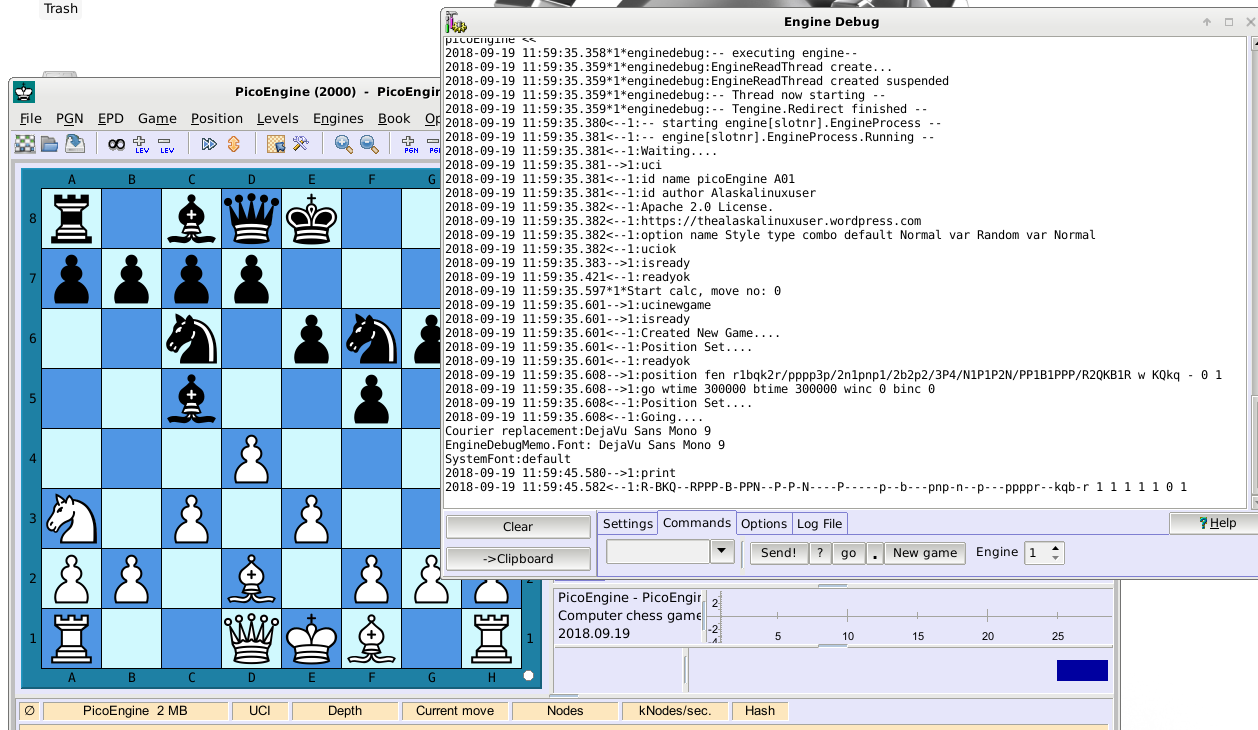One of the more interesting parts to programming chess engines, is using the UCI, Universal Chess Interface. In that interface standard are very specific settings. One of those is that engines should accept input as either move by move, or through FEN.

The FEN part was actually quite easy. Just breaking down characters of the line and figuring out what goes where. The toughest part was understanding vectors so I could do what I wanted to do. Here’s a glimps of the code:

vector<string> split(string str, char delimiter) {
vector<string> internal;
stringstream ss(str); // Turn the string into a stream.
string tok;

while(getline(ss, tok, delimiter)) {
internal.push_back(tok);
}

return internal;
}
………………………………………..Edited for space…………………………………
void inputPosition(string posString)
{
vector<string> sepVect = split(posString, ‘ ‘);
string str1 = sepVect;
if (sepVect == “fen”)
{
// FEN board setup.
string stringBoard = “”;
string str2 = sepVect;
for (int i = 0; (unsigned)i < str2.length(); i++) {
char myChar = str2.at(i);
if (isdigit(myChar))
{ int imyChar = myChar – ‘0’;
for (int a = 0; a < imyChar; a++)
{ stringBoard = stringBoard + “-“; }
}
else if (isalpha(myChar))
{ stringBoard = stringBoard + myChar; }
} // end for.
int j = stringBoard.length();
for (int i = 0; i < j; i++) {
if (i < 64){ theBoard[i] = stringBoard.at(i); }
}
if (sepVect.at(0) == ‘w’)
{ whitesTurn = true; } else { whitesTurn = false; }
if (sepVect.at(0) == ‘K’)
{ Kcastle = true; } else { Kcastle = false; }
if (sepVect.at(1) == ‘Q’)
{ Qcastle = true; } else { Qcastle = false; }
if (sepVect.at(2) == ‘k’)
{ kcastle = true; } else { kcastle = false; }
if (sepVect.at(3) == ‘q’)
{ qcastle = true; } else { qcastle = false; }
if (sepVect.at(0) == ‘-‘)
{ enPassant = false;} else { enPassant = true;}
moveSince = stoi (sepVect);
turnCount = stoi (sepVect);
} // End FEN

I just broke down the string into vectors separated by spaces. Then in each vector, I figured out where the piece needed to go, or how many blank spaces I needed.

Now I still have to work out a few oddities, but I think this should work fairly well, and seems to have in the few test runs I’ve given it. You can check out the whole commit on my GitLab if you’d like!

Linux – keep it simple.